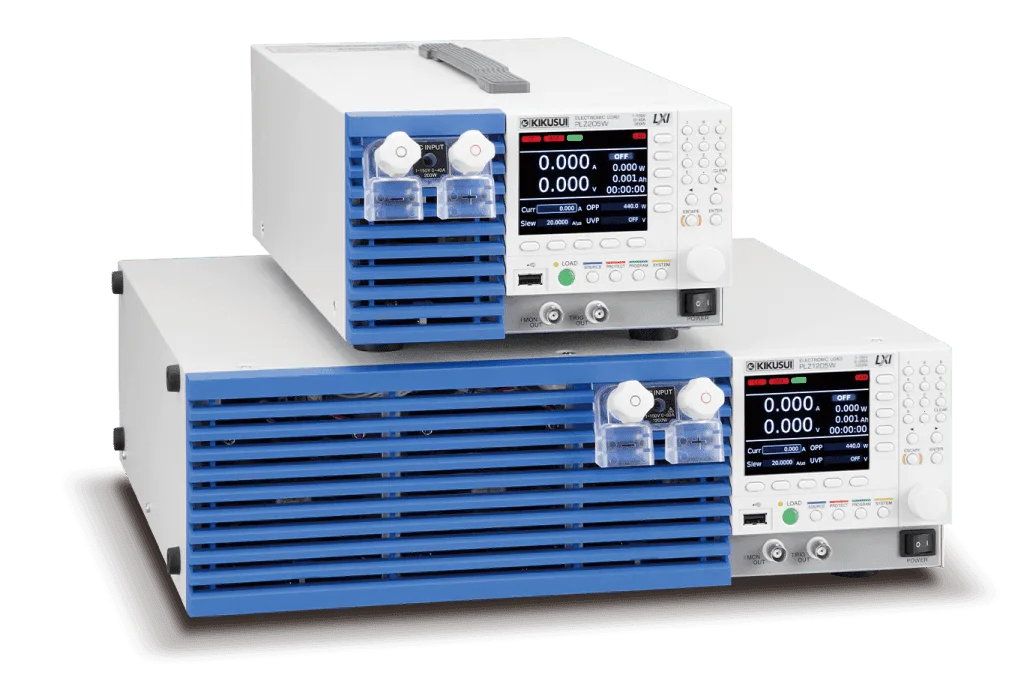### Various operations not possible with variable resistors are possible.

When measuring the characteristics of a device that outputs energy, such as a power supply or battery, a load that consumes energy is required. Variable resistors can be used for simple loads, but they may not be suitable for loads with complex behaviors. Electronic load devices are devices that use semiconductors such as transistors instead of variable resistors. Electronic load devices using semiconductors can be used as a variety of loads by incorporating a control circuit, since the current and voltage can be varied freely.• Arbitrary I-V characteristics: Installed “ARB mode”
• PLZ-5WZ Series Models with Impedance Measurement Function

• 200W, 400W, 1200W, 3 models
• Operation voltage: 0.25V to 150V (from 0.05V)
• High speed slew rate: 60A/µs
• Arbitrary I-V characteristics: Installed “ARB mode”
• Parallel operation feature: The total current and power capacities can be increased to the maximum of 10.8kW (2160A) by connecting the booster units.
• New high visibility color display.
• PLZ-5WZ Series Models with Impedance Measurement Function

• Ultra Compact
• Parallel operation: 5 units (Max. 100kW/ 2000A

#### Compact High VoltageDC Electronic Load

• Operating voltage: 10V to 1000V (Min. 1.5V)
• Power capacity: 1kW / 2kW / 4kW / 12kW / 20kW(5 models)
• 100 kW/ 2000 A with parallel operation (Max. 5 units), connectable with different models.
• LAN (LXI Compliant) / USB / RS232C standard interface *GPIB optional
• Synchronized operation / Sequence function
• Arbitrary IV characteristic (ARB) mode

• High-speed operation at 10µs
• Support of 0-V input

• Four different power ratings – 165W, 330W, 660W, and 1000W – five models in total
• Support of 0-V input (PLZ164WA and PLZ664WA)
• High-speed response supporting a maximum slew rate of 16A/µs (equivalent to 10µs when converted to a rise time)
• Support of constant current, constant resistance, constant voltage, constant power, constant current + constant voltage, and constant resistance + constant voltage modes
• Timer functions combined with time/voltage measurement functions enable battery discharge characteristic evaluations.
• Features sequence and switching functions.

• Full-Load Current of 100 A at 0.3 V!

• Two models, 165W and 330W
• Full-Load Current of 100A at 0.3V!(PLZ334WL)
• Possible to operate as low as 50mV of the input voltage
• Realize the fast slew rate of 50 A/μs at 2.3V of the load input terminal voltage. (Rise/Fall time conversion: Approx. 2 µs)
• Current setting resolution: 50µA (L range) *PLZ334WL
• 6 operation modes (CC, CR, CV, CP, CC+CV, CR + CV)
• Elapsed Time Display function and Auto Load- Off Timer function are convenient for the discharge tests of batteries
• Equipped with Sequence function and Switching function

• Parallel operation of load units
• Input operating voltage 0V model

• High-speed response supporting a maximum slew rate of 2.4 A/µs (PLZ150U)
• 0-V input operating voltage model (PLZ70UA)
• Up to 5 load units of the same model can be operated in parallel.
• Timer functions combined with time/voltage measurement functions enable battery discharge characteristic evaluations.
• Equipped with Sequence function and Switching function
• GPIB/RS232C are standard interface

• AC

• 1000W, 14V to 280V(rms), 0 to 10A(rms),45 to 65Hz
• Equipped with 3 operation modes, Constant Current, Constant Resistance, and Constant Power.
• Actual load simulation is possible with optional software.
• Up to 5 units can be operated in parallel Max. 5kW, 50Arms
• Equipped with tracking operation function
• Crest Factor Function